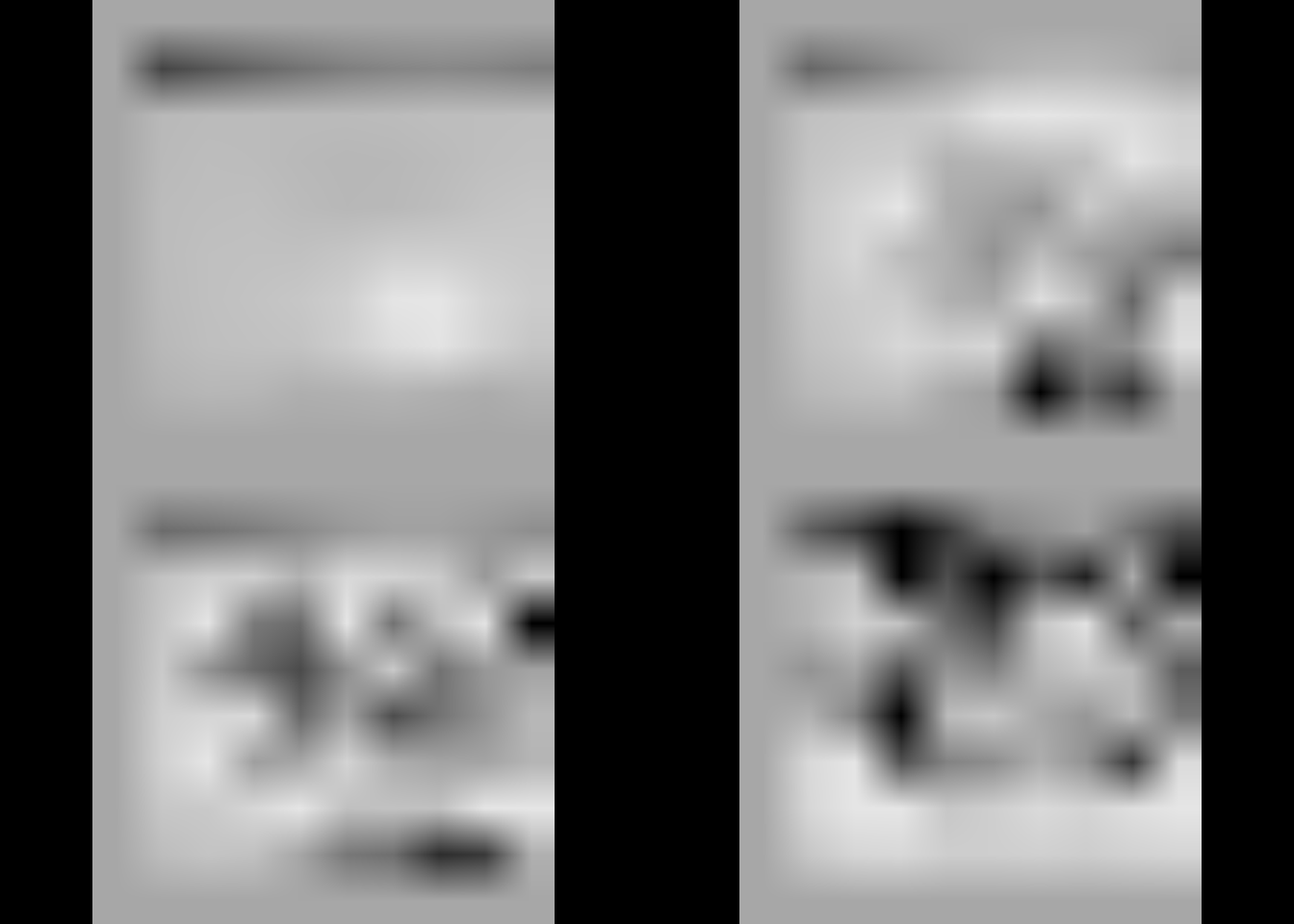The vast majority of problems tackled by RMINC as of 2016 are considered “embarassingly parallel” this means that the problem can be broken into smaller pieces that can be solved completely independently. Take for example the standard massively-univariate approach to analyzing neuroimages. Each image is composed of voxels, and a separate model is computed at each voxel. These models are computed without any dependency on the models being computed at other voxels. Since there is no interdependency it is easy to split the voxels up between multiple cores on a computer, or even between different computers and combine the results after.

## A Basic Example

Imagine for example there are some simple images that are 10 x 10 x 10 voxels.

``````library(RMINC)
suppressPackageStartupMessages(library(dplyr))

## Pull in some example data
getRMINCTestData("./")

## Read a csv containing the paths to the test files and some covariates
image_frame <- read.csv("rminctestdata/test_data_set.csv", stringsAsFactors = FALSE)

## Take a look at a few sample slices through the first volume volume
image_frame\$jacobians_fixed_2 %>%
mincGetVolume %>%
mincArray %>%
mincPlotSliceSeries(mfrow = c(2,2), begin = 2, end = -2)``````Now to see if the values at each voxel depend on some variable, body weight for example, we can fit a linear model. Since the models are independent we can divide the problem up. I’ll use four cores on my computer, each one will fit 250 of the 1000 models, and then the results can be stuck back together.

``model <- mincLm(jacobians_fixed_2 ~ Body.Weight, data = image_frame, parallel = c("local", 4))``
``## Range: 4.000000 1.000000``
``````image_frame\$jacobians_fixed_2 %>%
mincGetVolume %>%
mincArray %>%
mincPlotSliceSeries(statistic = mincArray(model, "tvalue-Body.Weight")
, symmetric = TRUE
, low = 2, high = 5
, begin = 2, end = -2
, legend = "t-value", locator = FALSE
, mfrow = c(2,2))``````